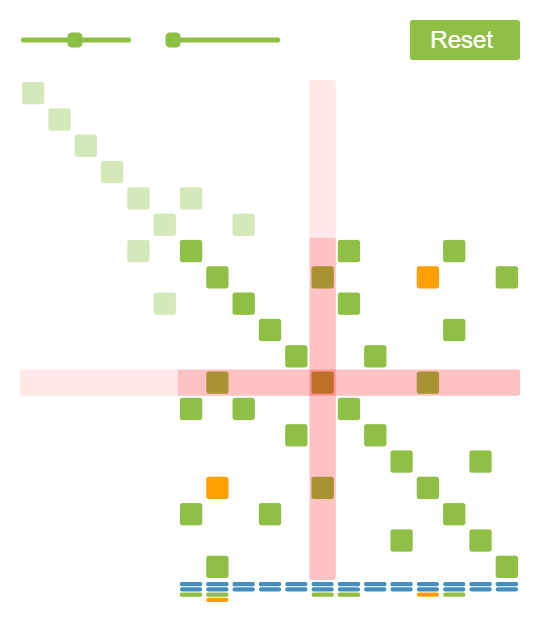# 最小二乘问题（六）

$\min \sum w_i\|r_i\|^2.$

$\min \sum \rho_i(\|r_i\|).$

\begin{aligned} \frac{\partial \|r\|^2}{\partial x} &= 2r^T\frac{\partial r}{\partial x} = 0 \\ \frac{\partial \rho(\|r\|)}{\partial x} &= \frac{d\rho}{dx} \frac{1}{\|r\|}r^T\frac{\partial r}{\partial x} = 0. \end{aligned}

$\frac12\frac{\partial w’\|r\|^2}{\partial r} = w’r^T\nabla r.$

# Cholesky 分解（番外二）# 最小二乘问题（五）

• 它有标准方程么？是什么形式？
• 它的解如何寻找？可以求解标准方程么？
• 它有全局最优解么？

$r(x_0+\Delta x) = r(x_0)+J_r\Delta x.$

$\min_{\Delta x} \|J_r\Delta x + r(x_0)\|^2.$

$J_r^TJ_r\Delta x = -J_r^Tr(x_0).$

1. $x \gets x_0;$
2. $\Delta x \gets \arg\min_{\Delta x} |J_r\Delta x+r(x)|^2;$
• 求解标准方程 $J_r^TJ_r\Delta x = -J_r^Tr(x);$
3. $x \gets x+\gamma \Delta x;$
4. 回到 2 ，除非 $|\Delta x|<\epsilon$ 。

# Photo